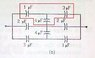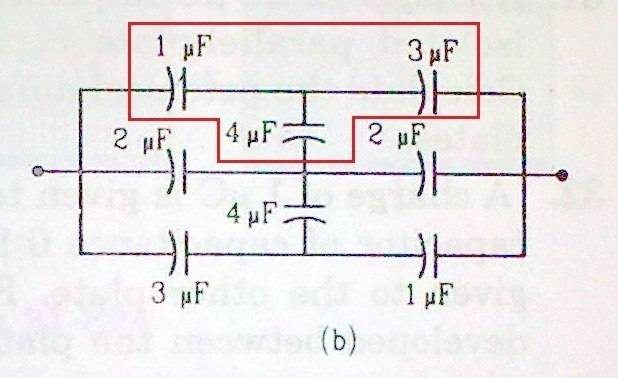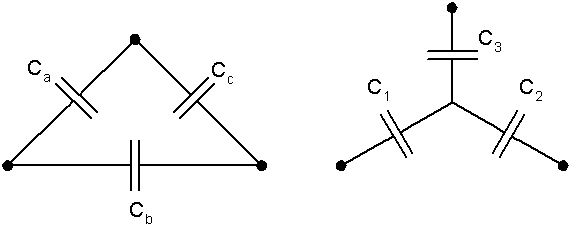# Y-Delta conversion for Capacitors

cupid.callin
This was a ques in my book ... (Pic)

After thinking a while, i realized that it can be solved using Y-Delta (by converting Y to delta form) conversion. But i couldn't get the answer ... i don't know why ...

I used this http://en.wikipedia.org/wiki/Y-%CE%94_transform" [Broken]

It is given for resistors ... i guess its same for resistors and capacitors ... Right?

Please tell me if the eqn used is wrong or something else!

#### Attachments

•Y-Delta.jpg
25.4 KB · Views: 17,693
Last edited by a moderator:

Mentor
This was a ques in my book ... (Pic)

After thinking a while, i realized that it can be solved using Y-Delta (by converting Y to delta form) conversion. But i couldn't get the answer ... i don't know why ...

I used this http://en.wikipedia.org/wiki/Y-%CE%94_transform" [Broken]

It is given for resistors ... i guess its same for resistors and capacitors ... Right?

Please tell me if the eqn used is wrong or something else!

Remember how capacitors combined differently in series and parallel than do resistors?

What you can do is convert all your capacitances to their equivalent impedance, then use those formulae. Impedances mix and match like resistances.

Last edited by a moderator:
cupid.callin
How do i find impedance of a capacitor?

Mentor
How do i find impedance of a capacitor?

$$Zc = 1/(j\omega C$$)

$$\omega$$ is the operating frequency. The result is in Ohms, and will be an imaginary value.

Don't panic! You don't need to know the frequency for the math to work out; it's a constant for the given Y to Delta situation. If you do the algebra, a pretty simple result obtains. If the resistor version is:

Ra = (R1*R2 + R2*R3 + R3*R1)/R2
Rb = (R1*R2 + R2*R3 + R3*R1)/R3
Rc = (R1*R2 + R2*R3 + R3*R1)/R1

Then the capacitor version looks like:

Ca = C1*C3/(C1 + C2 + C3)
Cb = C1*C2/(C1 + C2 + C3)
Cc = C2*C3/(C1 + C2 + C3)

cupid.callin
And to find Y form,

C1 = (CaCb + CbCc + CcCa) / Cc
Right?

Thanks for the help gneill !
Thanks a lot !

Mentor
And to find Y form,

C1 = (CaCb + CbCc + CcCa) / Cc
Right?

Thanks for the help gneill !
Thanks a lot !

Right.

You're welcome.

Staff Emeritus
Homework Helper
Gold Member
... for the given Y to Delta situation. If you do the algebra, a pretty simple result obtains. If the resistor version is:

Ra = (R1*R2 + R2*R3 + R3*R1)/R2
Rb = (R1*R2 + R2*R3 + R3*R1)/R3
Rc = (R1*R2 + R2*R3 + R3*R1)/R1

Then the capacitor version looks like:

Ca = C1*C3/(C1 + C2 + C3)
Cb = C1*C2/(C1 + C2 + C3)
Cc = C2*C3/(C1 + C2 + C3)The three capacitors enclosed in red form a Y. So does the other set of 1, 3, and 4 μF capacitors. Converting each of these sets to Δ configuration, as shown by gneill above, will allow you analyze the circuit as a combination of parallel and series capacitors.

cupid.callin
Thanks for the help!

PriyankB
Is there a simple result for delta to Y as well?

Mentor
Is there a simple result for delta to Y as well?

Sure. Just substitute the appropriate capacitor impedances into the formulas for resistance, stir and serve. Note that a capacitor impedance is of the form $1/(j \omega C)$.So for example, given that for resistors
$$R_1 = \frac{R_a R_b}{R_a + R_b + R_c}$$
then
$$\frac{1}{C_1} = \frac{C_c}{C_b C_c + C_a C_c + C_a C_b}$$

and so on.

EDIT: Fixed up the expression. Should have been 1/C1 on the LHS.

Last edited:
PriyankB
Thank you! :)